Home > Processing > Processing Code Snippits

# Processing Code Snippets

Processing is an open source programming language and environment for people who want to create images, animations, and interactions. Initially developed to serve as a software sketchbook and to teach fundamentals of computer programming within a visual context, Processing also has evolved into a tool for generating finished professional work. Today, tens of thousands of students, artists, designers, researchers, and hobbyists who use Processing for learning, prototyping, and production.

https://github.com/gwygonik/SquiggleDraw/tree/master/SquiggleDraw

## Create a pallet from an image

```// Code borrowed from
// http://www.complexification.net/gallery/machines/substrate/index.php
// j.tarbell   April, 2005

// Pallet parameters
int maxpal = 812;
int numpal = 0;
color[] goodcolor = new color[maxpal];
int size = 15;
int count;

void setup() {
size(550, 600);
noLoop();
}

void draw() {
background(0);
pickColourFromPallet("pallet.png");

// show pallet colours
for (int i = 0; i < 30; i ++) {
for (int j = 0; j < maxpal / 30; j ++) {
ellipse(size + (i * size), j * size + 170, size, size);
fill(goodcolor[count]);
count ++;
}
}
}

void pickColourFromPallet(String fn) {
PImage b;
image(b, 0, 0);

for (int x=0; x<b.width; x++) {
for (int y=0; y<b.height; y++) {
color c = get(x, y);
boolean exists = false;
for (int n=0; n<numpal; n++) {
if (c==goodcolor[n]) {
exists = true;
break;
}
}
if (!exists) {
if (numpal<maxpal) {
goodcolor[numpal] = c;
numpal++;
}
}
}
}
}```

### Save screen with key click

// Save the screen as a png file when you press the s key exit if you type x
// note requires a global variable called click

void keyTyped() {
println("typed " + int(key) + " " + keyCode);

if (int(key) == 115) { // s for save
save("circle005-" + click + ".png");
println("Saved: " + "circle005-" + click + ".png");
click ++;
}

if (int(key) == 120) {
exit(); // x to exit the program
}
}

### Draw n points on a circle

```void setup() {
background(220);
size(300, 300);
noLoop();
}

void draw() {
// Draw n points around a circle
float h = width / 2;
float k = height / 2;
float degree = 0;
float numberOfPoints = 37; // note has to be a float
float circleRadius = width / 2.5;
for (int i = 0; i < numberOfPoints; i++) {

println(i);
degree = degree + 360 / numberOfPoints;
}
save("points.png");
}

```### How to calculate points on a circle

// How to calculate points on a circle
// Based on code from http://www.mathopenref.com/coordcirclealgorithm.html

void setup() {
background(255);
strokeWeight(4); // Thicker
size(300, 300);
smooth(8);
}

void draw() {
double step = 2 * PI/20; // see note 1
float h = 150;
float k = 150;
float r = 50;

for(float theta=0; theta < 2 * PI; theta += step) {
float x = h + r * cos(theta);
float y = k - r * sin(theta); //note 2.
point(x,y);
}
}

/*
Like most graphics systems, the canvas element differs from the usual mathematical coordinate plane:

The origin is in the top left corner.
The code above compensates by assuming that h, and k are actually relative to the top left.
The y axis is inverted. Positive y is down the screen, not up. To correct for this, the k variable (the y coordinate of the center) must be positive to place the center some way down the screen.

Also the y calculation has to subtract the sin(x) term instead of add.
Marked in the code as Note 1.

Note 2. The step size is set to an exact division of 2π to avoid gaps or over-runs in the circle. This code divides the circle into exactly 20 segments.
Note too that as in most computer languages, the trig functions operate in radians, not degrees.
*/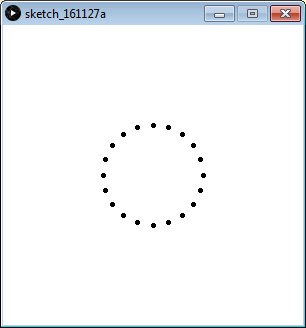### Draw an Arc between X,Y points on a circle

stroke(255,0,0);
strokeWeight(5);
arc(0, 0, 590, 590, atan2(y3, x3), atan2(y5, x5));
arc(0, 0, 590, 590, atan2(y9, x9), atan2(y10, x10));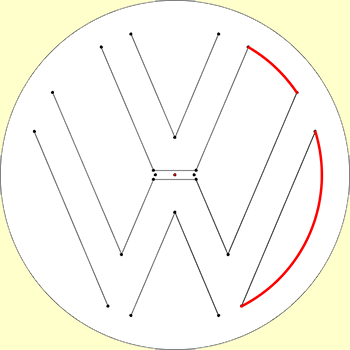### Text along a curve

// The message to be displayed
String message = "text along a curve";

PFont f;
// The radius of a circle
float r = 100;

void setup() {
size(320, 320);
f = createFont("Georgia",40,true);
textFont(f);
// The text must be centered!
textAlign(CENTER);
smooth();
}

void draw() {
background(255);

// Start in the center and draw the circle
translate(width / 2, height / 2);
noFill();
stroke(0);
ellipse(0, 0, r*2, r*2);

// We must keep track of our position along the curve
float arclength = 0;

// For every box
for (int i = 0; i < message.length(); i++)
{
// Instead of a constant width, we check the width of each character.
char currentChar = message.charAt(i);
float w = textWidth(currentChar);

// Each box is centered so we move half the width
arclength += w/2;
// Starting on the left side of the circle by adding PI

float theta = PI + arclength / r;

pushMatrix();
// Polar to cartesian coordinate conversion
translate(r*cos(theta), r*sin(theta));

// Rotate the box
rotate(theta+PI/2); // rotation is offset by 90 degrees
// Display the character

fill(0);
text(currentChar,0,0);
popMatrix();
// Move halfway again
arclength += w/2;
}
}//https://amnonp5.wordpress.com/2012/01/28/25-life-saving-tips-for-processing/
//16. high resolution output
//Sometimes you only need to draw to the screen. Sometimes you want to save that output.
//Sometimes you need to have that output in a very high resolution, for example when you
//want to print it. There are different ways to generate high resolution output.
//One can use the PDF export options. Or one could use one of the many different ‘hacks’
//to create a high resolution copy of the regular draw() loop.
//Here is an example of one such technique. void setup() {
size(100, 100);
}

void draw() {
noLoop();
background(255);
smooth();
strokeWeight(0.5);

for (int x = 0; x < 500; x ++) {
fill(random(255), random(255), random(255));
float size =  random(1, 10);
ellipse(random(100), random(100), size, size);
}
}

void keyPressed() {
if (key == 's') {
save("normal.png");
saveHiRes(10);  // saves a copy @ 5000 * 5000 pixels
exit();
}
}

void saveHiRes(int scaleFactor) {
println("starting to save high.res copy");
PGraphics hires = createGraphics(width*scaleFactor, height*scaleFactor, JAVA2D);
beginRecord(hires);
hires.scale(scaleFactor);
draw();
endRecord();
hires.save("hires2.png");
println("saved high.res copy");
exit();
}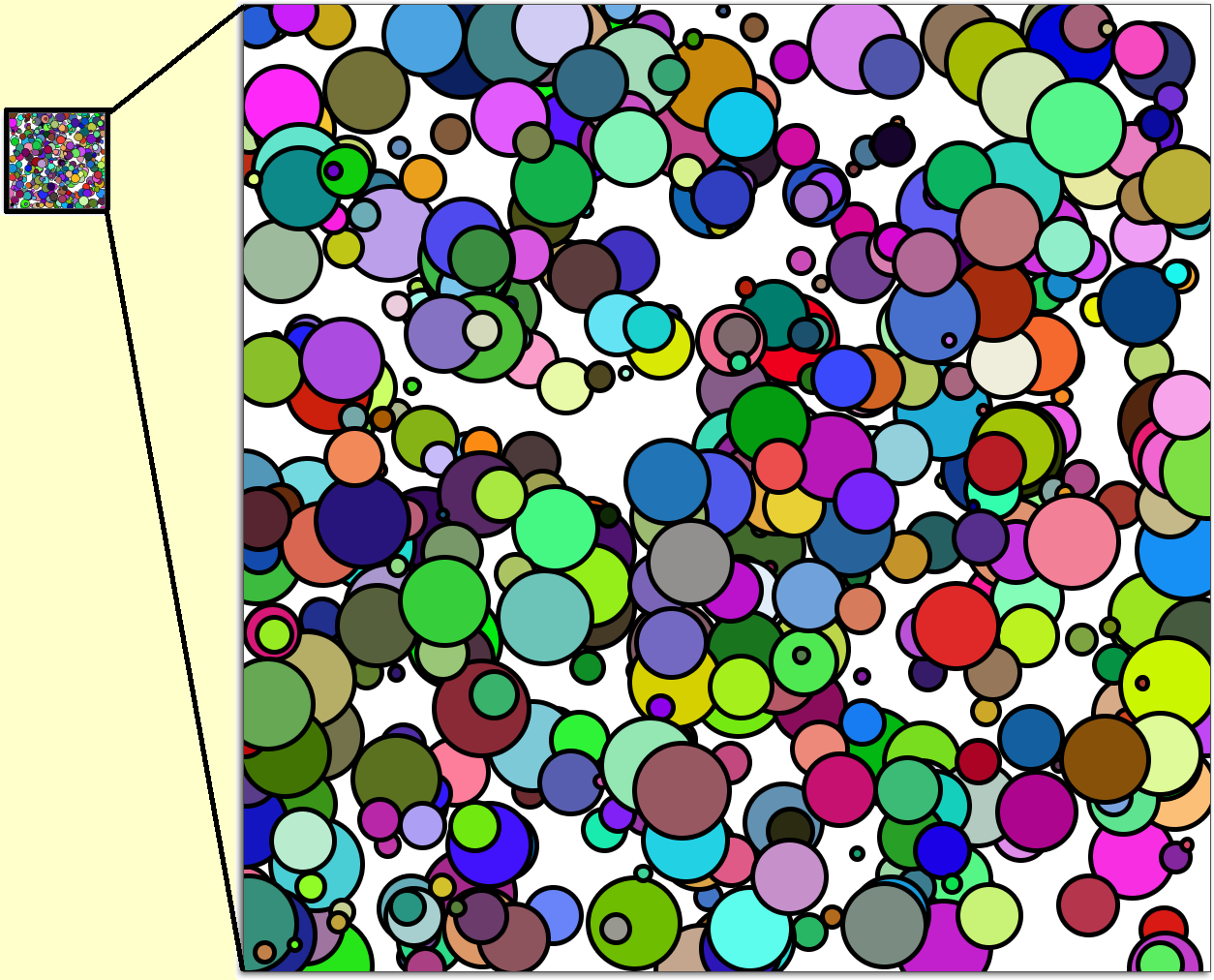### Change pixel colours in an image

PImage img; //declare the image
void setup() {
size(300, 300);

color checkColor = color(#000000); //the color to check for
color setColor = color(#FFFF00); //the color to replace it with (Yellow in this example)

//pixel array method
for (int p=0; p<img.width*img.height; p++) {
//loop through all the pixels
if (img.pixels[p]==checkColor) {
//if the pixel has the color we are checking for
img.pixels[p]=setColor; //replace it with the new color
}
}
img.updatePixels(); //then update the pixels
image(img, 0, 0); //now show the image
save("new-grid.gif");
}BecomesAll black pixels are replaced with yellow ones

### Round Numbers and convert to strings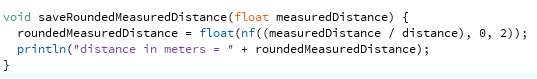roundedMeasuredDistance = float(nf((measuredDistance / distance), 0, 2));

float f1 = 1.2; println(nf(f1, 0, 2)); // 1.20
float f2 = 1.234; println(nf(f2, 0, 2)); // 1.23
float f3 = 1.0; println(nf(f3, 0, 2)); // 1.00
float f4 = 1234.567; println(nf(f4, 0, 2)); // 1234.57 - rounded upThanks to Daniel Shiffman

http://learningprocessing.com/examples/chp15/example-15-07-PixelArrayImage#becomes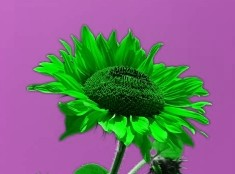Note code formatting by Processing > EDIT > Copy as HTML

```// Example 15-7: Displaying the pixels of an image
// http://learningprocessing.com/examples/chp15/example-15-07-PixelArrayImage#

PImage img;

void setup() {
size(235, 174);
}

void draw() {

// We must also call loadPixels() on the PImage since we are going to read its pixels.
for (int y = 0; y < height; y++ ) {
for (int x = 0; x < width; x++ ) {
int loc = x + y*width;
// The functions red(), green(), and blue() pull out the three color components from a pixel.
float r = red(img.pixels [loc]);
float g = green(img.pixels[loc]);
float b = blue(img.pixels[loc]);

// Image Processing would go here
// If we were to change the RGB values, we would do it here, before setting the pixel in the display window.

// Set the display pixel to the image pixel
pixels[loc] = color(b, g, b);
}
}

updatePixels();
}

void mouseClicked(){

save("new sunflower.jpg");

}

```
```

// Generate a random angle
int angle;
int case_0;
int case_1;
int case_2;
int case_3;
int case_4;
int case_5;
int case_6;
int case_7;
int case_8;

void draw() {
// Get a random element from an array
int index = int(random(8));

switch(index) {
case 0:
angle = 0;
case_0 ++;
break;
case 1:
angle = 45;
case_1 ++;
break;
case 2:
angle = 90;
case_2 ++;
break;
case 3:
angle = 135;
case_3 ++;
break;
case 4:
angle = 180;
case_4 ++;
break;
case 5:
angle = 225;
case_5 ++;
break;
case 6:
angle = 270;
case_6 ++;
break;
case 7:
angle = 315;
case_7 ++;
break;
default:
case_8 ++;
angle = 0;
break;
}

println("case_0 = " + case_0);
println("case_1 = " + case_1);
println("case_2 = " + case_2);
println("case_3 = " + case_3);
println("case_4 = " + case_4);
println("case_5 = " + case_5);
println("case_6 = " + case_6);
println("case_7 = " + case_7);
println("case_8 = " + case_8);
}

// Get a random element from an array
String[] words = { "apple", "bear", "cat", "dog" };
int index = int(random(words.length));  // Same as int(random(4))
println(words[index]);  // Prints one of the four words

int rand;
void draw() {
// Get a random element from an array
int[] angles = { 0, 45, 90, 135, 180, 255, 270, 315};
int index = int(random(angles.length));  // Same as int(random(4))
println(angles[index]);  // Prints one of the eight angles
}

Collision Detection Ellipse / Ellipse

// http://jeffreythompson.org/collision-detection/circle-circle.php

float c1x = 0;      // circle 1 position
float c1y = 0;      // (controlled by mouse)
float c1r = 30;     // radius

float c2x = 300;    // circle 2 position
float c2y = 200;
float c2r = 100;

void setup() {
size(600,400);
noStroke();
}

void draw() {
background(255);

// update position to mouse coordinates
c1x = mouseX;
c1y = mouseY;

// check for collision
// if hit, change color
boolean hit = circleCircle(c1x,c1y,c1r, c2x,c2y,c2r);
if (hit) {
fill(255,150,0);
}
else {
fill(0,150,255);
}
ellipse(c2x,c2y, c2r*2,c2r*2);

// other circle, controlled by mouse
fill(0, 150);
ellipse(c1x,c1y, c1r*2,c1r*2);
}

// CIRCLE/CIRCLE
boolean circleCircle(float c1x, float c1y, float c1r, float c2x, float c2y, float c2r) {

// get distance between the circle's centers
// use the Pythagorean Theorem to compute the distance
float distX = c1x - c2x;
float distY = c1y - c2y;
float distance = sqrt( (distX*distX) + (distY*distY) );

// if the distance is less than the sum of the circle's
// radii, the circles are touching!
if (distance <= c1r+c2r) {
return true;
}
return false;
}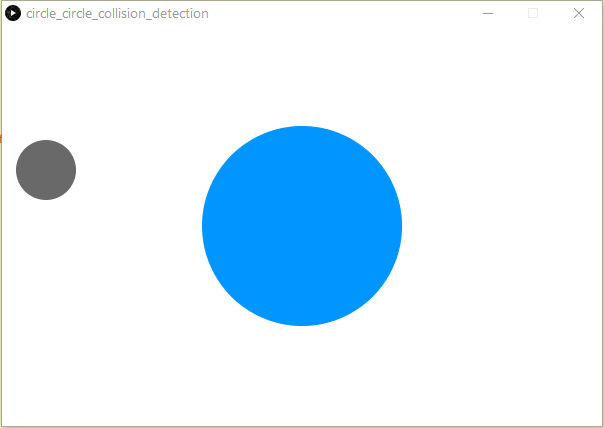```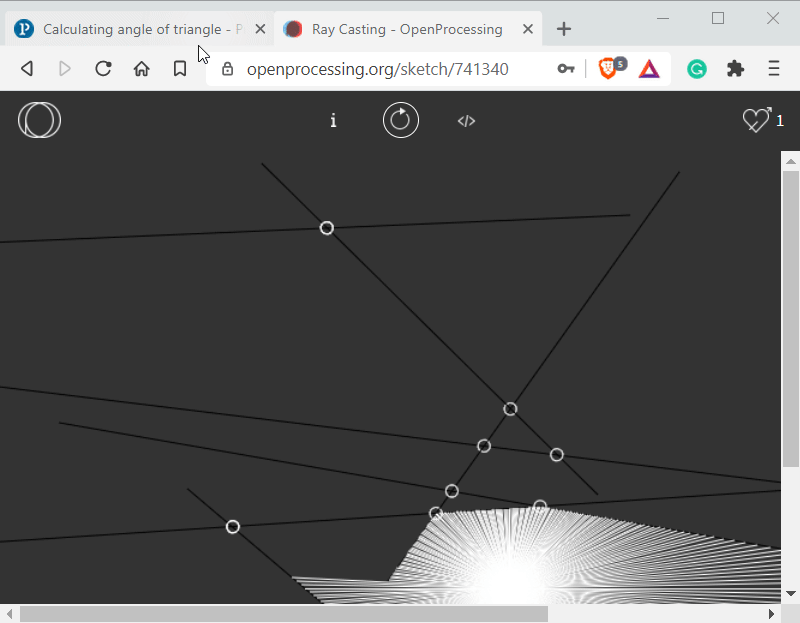https://www.openprocessing.org/sketch/741340

A book on collision detection with Processing

https://jeffreythompson.org/collision-detection/line-line.php

APA citation:
Russell, R. (2020, October 14, 05:35 am). Processing code snippets.
Retrieved June 01, 2023, from
http://www.rupert.id.au/processing/snippets.php

Last refreshed: June 01 2023. 04:16.17 pm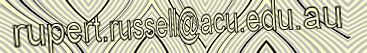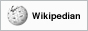This work is licensed under a Creative Commons Attribution-NonCommercial-ShareAlike 2.5 License.

746 Visits since November 17, 2016